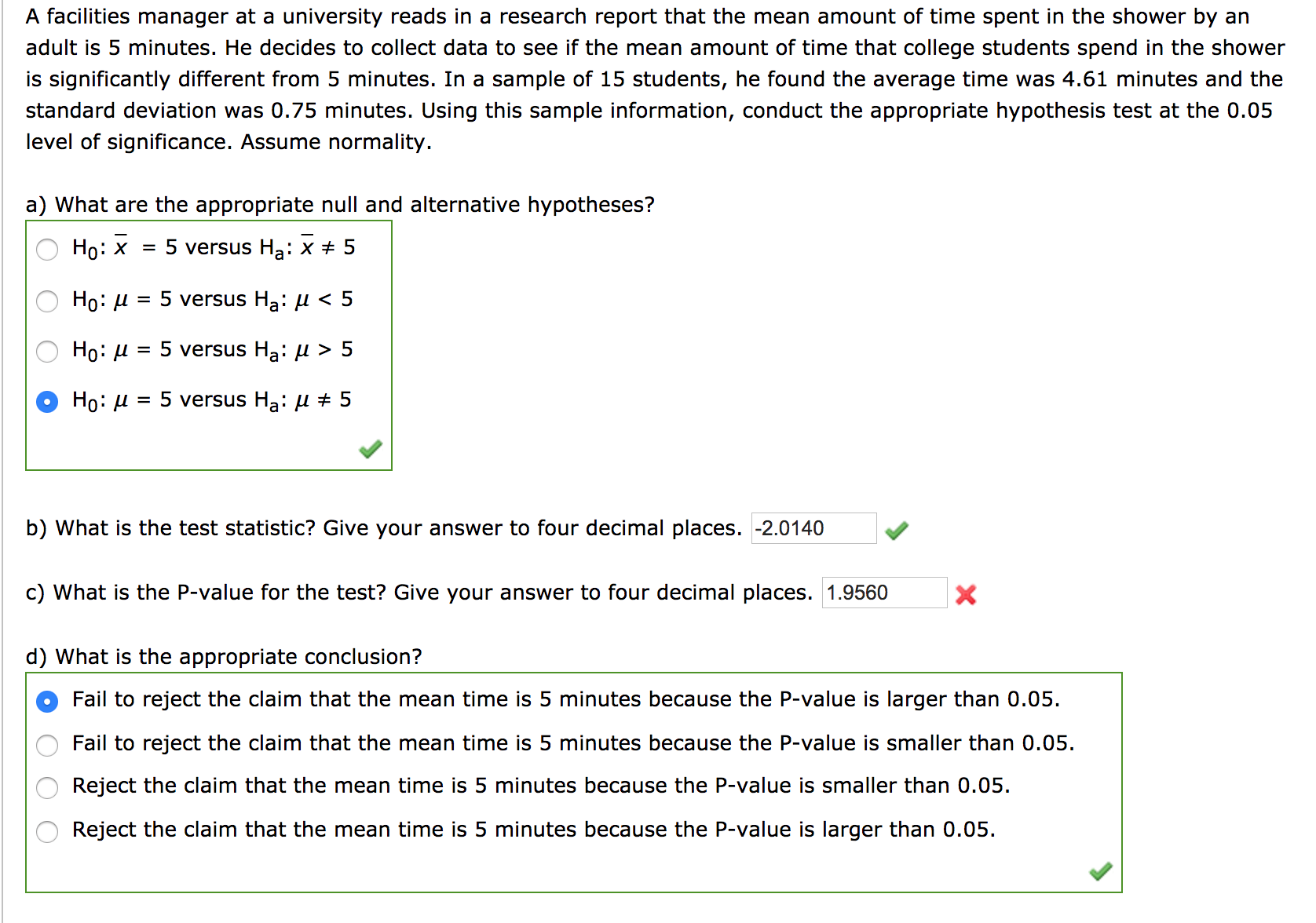# A facilities manager at a university reads in a research report that the mean amount of time spent in the shower by an adult is 5 minutes. He decides to collect data to see if the mean amount of time that college students spend in the shower is significantly different from 5 minutes. In a sample of 15 students, he found the average time was 4.61 minutes and the standard deviation was 0.75 minutes. Using this sample information, conduct the appropriate hypothesis test at the 0.05 level of significance. Assume normality. a) What are the appropriate null and alternative hypotheses? Но: х = 5 versus Ha: x # 5 5 versus Ha: H< 5 Но: и 5 versus Ha: l > 5 Но: и Но: и 5 versus Ha: l # 5 b) What is the test statistic? Give your answer to four decimal places. -2.0140 c) What is the P-value for the test? Give your answer to four decimal places. 1.9560 X d) What is the appropriate conclusion? Fail to reject the claim that the mean time is 5 minutes because the P-value is larger than 0.05 Fail to reject the claim that the mean time is 5 minutes because the P-value is smaller than 0.05. Reject the claim that the mean time is 5 minutes because the P-value is smaller than 0.05 Reject the claim that the mean time is 5 minutes because the P-value is larger than 0.05

Questionhelp_outlineImage TranscriptioncloseA facilities manager at a university reads in a research report that the mean amount of time spent in the shower by an adult is 5 minutes. He decides to collect data to see if the mean amount of time that college students spend in the shower is significantly different from 5 minutes. In a sample of 15 students, he found the average time was 4.61 minutes and the standard deviation was 0.75 minutes. Using this sample information, conduct the appropriate hypothesis test at the 0.05 level of significance. Assume normality. a) What are the appropriate null and alternative hypotheses? Но: х = 5 versus Ha: x # 5 5 versus Ha: H< 5 Но: и 5 versus Ha: l > 5 Но: и Но: и 5 versus Ha: l # 5 b) What is the test statistic? Give your answer to four decimal places. -2.0140 c) What is the P-value for the test? Give your answer to four decimal places. 1.9560 X d) What is the appropriate conclusion? Fail to reject the claim that the mean time is 5 minutes because the P-value is larger than 0.05 Fail to reject the claim that the mean time is 5 minutes because the P-value is smaller than 0.05. Reject the claim that the mean time is 5 minutes because the P-value is smaller than 0.05 Reject the claim that the mean time is 5 minutes because the P-value is larger than 0.05 fullscreen

### Want to see the step-by-step answer?